Question

# A cable lowers a 1400 kg elevator so that the elevator's speed increases from zero to...

A cable lowers a 1400 kg elevator so that the elevator's speed increases from zero to 4.0 m/s in a vertical distance of 5.6 m .

What is the force that the cable exerts on the elevator while lowering it? (Assume that the system is the elevator and Earth.)

What is the force that the cable exerts on the elevator while lowering it? (Assume that the system is the elevator only.)

here,

initial speed , u = 0

final speed , v = 4 m/s

vertical distance , h = 5.6 m

let the accelration be a

v^2 - u^2 = 2 * a * h

4^2 - 0 = 2 * a * 5.6

a = 1.43 m/s^2

let the tension in the rope be t

m * g - t = m * a

1400 * 9.8 - t = 1400 * 1.43

t = 11718 N

the force that the cable exerts on the elevator while lowering it is 11718 N for both parts

A cable lowers a 1300 $\mathrm{k}\mathrm{g}$ elevator so that the elevator's speed increases from zero to 4.0 $\mathrm{m}/\mathrm{s}$ in a vertical distance of 6.4 $\mathrm{m}$.

#### Earn Coins

Coins can be redeemed for fabulous gifts.

Similar Homework Help Questions
• ### A cable lowers a 1150 kg elevator so that the elevator's speed increases from zero to...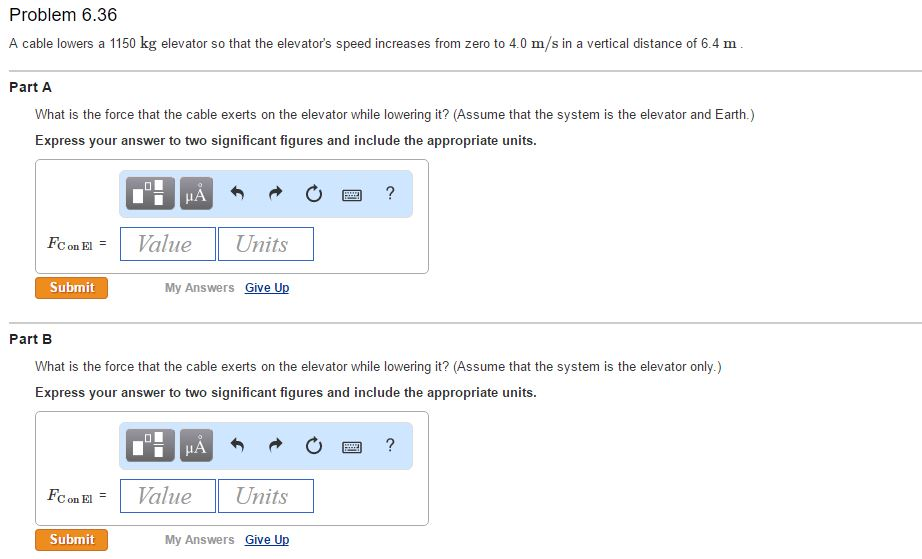A cable lowers a 1150 kg elevator so that the elevator's speed increases from zero to 4.0 m/s in a vertical distance of 6.4 m What is the force that the cable exerts on the elevator while lowering it? (Assume that the system is the elevator and Earth.) Express your answer to two significant figures and include the appropriate units. What is the force that the cable exerts on the elevator while lowering it? (Assume that the system is the...

• ### A fully loaded, slow-moving freight elevator has a cab with a total mass of 1300 kg,...

A fully loaded, slow-moving freight elevator has a cab with a total mass of 1300 kg, which is required to travel upward 47 m in 3.5 min, starting and ending at rest. The elevator's counterweight has a mass of only 800 kg, so the elevator motor must help pull the cab upward. What average power is required of the force the motor exerts on the cab via the cable?

• ### An elevator is pulled upward so it moves with increasing upward speed?the force exerted by the...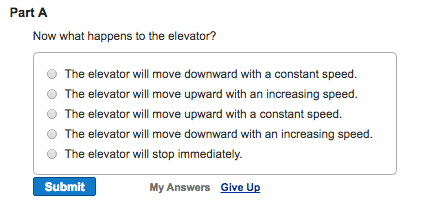An elevator is pulled upward so it moves with increasing upward speed?the force exerted by the cable on the elevator is constant and greater than the downward gravitational force exerted by Earth. When the elevator is moving up medium-fast, the force exerted by the cable on the elevator changes abruptly to just balance the downward gravitational force of Earth?the sum of the forces that the cable and Earth exert on the elevator is now zero.

• ### Chapter 07, Problem 049 A fully loaded, slow-moving freight elevator has a cab with a total...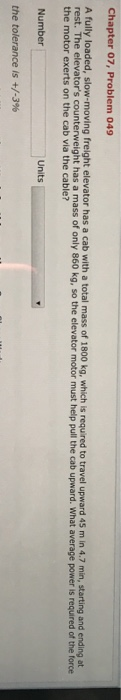Chapter 07, Problem 049 A fully loaded, slow-moving freight elevator has a cab with a total mass of 1800 kg, which is required to travel upward 45 m in 4.7 min, starting and ending at rest. The elevator's counterweight has a mass of only 860 kg, so the elevator motor must help pull the cab upward. What average power is required of the force the motor exerts on the cab via the cable? Number the tolerance is +/-3% Units

• ### Jumping up before the elevator hits. After the cable snaps and the safety system fails, an...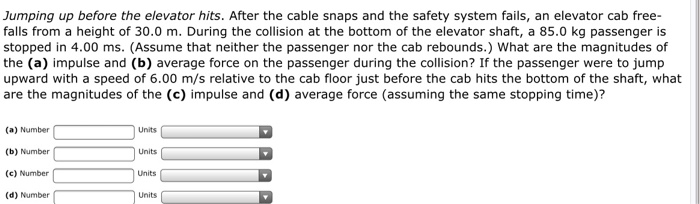Jumping up before the elevator hits. After the cable snaps and the safety system fails, an elevator cab free-falls from a height of 30.0 m. During the collision at the bottom of the elevator shaft, a 85.0 kg passenger is stopped in 4.00 ms. (Assume that neither the passenger nor the cab rebounds.) What are the magnitudes of the (a) impulse and (b) average force on the passenger during the collision? If the passenger were to jump upward with a...

• ### The cable of a crane is lifting a 850 kg girder. The girder increases its speed...

The cable of a crane is lifting a 850 kg girder. The girder increases its speed from 0.25 m/s to 0.75 m/s in a distance of 5.5 m. How much work is done by tension?

• ### An elevator has mass 600 kg, not including passengers. The elevator is designed to ascend, at...

An elevator has mass 600 kg, not including passengers. The elevator is designed to ascend, at constant speed, a vertical distance of 20.0 m (five floors) in 16.0 s, and it is driven by a motor that can provide up to 40 hp to the elevator. A. What is the maximum number of passengers that can ride in the elevator? Assume that an average passenger has mass 65.0 kg.

• ### An elevator has mass 650 kg , not including passengers. The elevator is designed to ascend,...

An elevator has mass 650 kg , not including passengers. The elevator is designed to ascend, at constant speed, a vertical distance of 21.0 m(five floors) in 16.7 s , and it is driven by a motor that can provide up to 44 hp to the elevator. What is the maximum number of passengers that can ride in the elevator? Assume that an average passenger has mass 65.0 kg.

• ### QUESTION 24 An elevator of total mass 200 kg is supported by a single cable, as...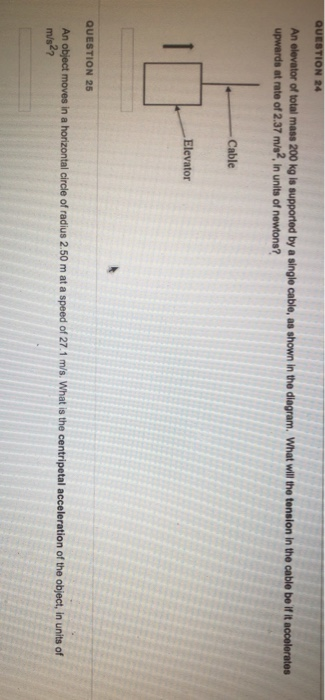QUESTION 24 An elevator of total mass 200 kg is supported by a single cable, as shown in the diagram. What will the tension in the cable be if it accelerates upwards at rate of 2.37 m/s2, in units of newtons? -Cable Elevator QUESTION 25 An object moves in a horizontal circle of radius 2.50 m at a speed of 27.1 m/s. What is the centripetal acceleration of the object, in units of m/s2?

• ### A single mass (m1 = 4.4 kg) hangs from a spring in a motionless elevator.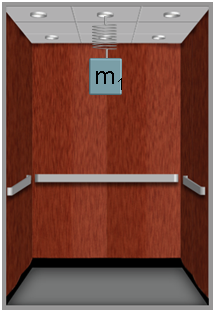A single mass (m1 = 4.4 kg) hangs from a spring in a motionless elevator. The spring constant is k = 270 N/m. 1) What is the distance the spring is stretched from its unstretched length?  2) Now, three masses (m1 = 4.4 kg, m2 = 13.2 kg and m3 = 8.8) hang from three identical springs in a motionless elevator. The springs all have the same spring constant given above. What is the magnitude of the force the bottom spring exerts on the...